## Database-Management-System

 Question 1

In a B+ tree, if the search-key value is 8 bytes long, the block size is 512 bytes and the block pointer size is 2 bytes, then the maximum order of the B+ tree is _________.

 A 52 B 53 C 54 D 55
Database-Management-System       B+-Trees       GATE 2017 [Set-2]       Video-Explanation
Question 1 Explanation:
Given,
Block size = 512 bytes
Block pointer = 2 bytes
Search key = 8 bytes
Let Maximum order of B+ tree = n
n(Pb) + (n – 1)(k) ≤ 512
n(2) + (n – 1)(8) ≤ 512
2n + 8n – 8 ≤ 512
10n ≤ 520
n ≤ 520/10
(n = 52)
 Question 2

Which one of the following statements is NOT correct about the B+ tree data structure used for creating an index of a relational database table?

 A Each leaf node has a pointer to the next leaf node B Non-leaf nodes have pointers to data records C B+ Tree is a height-balanced tree D Key values in each node are kept in sorted order
Database-Management-System       B-and-B+-Trees       GATE 2019       Video-Explanation
Question 2 Explanation:
Memory based question:
In B+ trees non-leaf nodes do not have pointers to data records.
 Question 3
Consider the following two statements about database transaction schedules:
I. Strict two-phase locking protocol generates conflict serializable schedules that are also recoverable.
II. Timestamp-ordering concurrency control protocol with Thomas Write Rule can generate view serializable schedules that are not conflict serializable.
Which of the above statements is/are TRUE?
 A Both I and II B Neither I nor II C II only D I only
Database-Management-System       Transactions       GATE 2019       Video-Explanation
Question 3 Explanation:
(Memory-based question)
In strict 2PL, a transaction T does not release any of its exclusive (write) locks until after it commits or aborts.
Hence, no other transaction can read or write an item that is written by T unless T has committed, leading to a strict schedule for recoverability.
(Ref: Fundamentals of Database Systems by Elmasri and Navathe, 7e Pg. No. 789)
By ignoring the write, Thomas write rule allows schedules that are not conflict serializable but are nevertheless correct.
Those non-conflict-serializable schedules allowed satisfy the definition of view serializable schedules.
(Ref: Database System Concepts by Silberschatch, Korth and Sudarshan, 6e Pg No. 686)
 Question 4
Consider the following relations P(X,Y,Z), Q(X,Y,T) and R(Y,V).How many tuples will be returned by the following relational algebra query?
x(P.Y=R.Y ∧ R.V=V2)(P × R)) – ∏x(Q.Y=R.Y ∧ Q.T>2)(Q × R))
 A 0 B 1 C 2 D 3
Database-Management-System       Relational-Algebra       GATE 2019       Video-Explanation
Question 4 Explanation:
σ(P.Y = R.Y ∧ R.V = V2)(P × R)x(P.Y = R.Y ∧ R.V = V2)(P × R))σ(Q.Y = R.Y ∧ Q.T>2)(Q × R)x(Q.Y = R.Y ∧ Q.T>2)(Q × R))x(P.Y = R.Y ∧ R.V = V2)(P × R)) – ∏x(Q.Y = R.Y ∧ Q.T>2)(Q × R))Question 5

A relational database contains two tables Student and Performance as shown below:The primary key of the Student table is Roll_no. For the Performance table, the columns Roll_no. and Subject_code together from the primary key. Consider the SQL query given below:

```SELECT S.Student_name, sum (P.Marks)
FROM Student S, Performance P
WHERE P.Marks > 84
GROUP BY S.Student_name;```

The number of rows returned by the above SQL query is _____.

 A 0 B 9 C 7 D 5
Database-Management-System       SQL       GATE 2019       Video-Explanation
Question 5 Explanation:
(Executed under Oracle Express Edition)
SQL> SELECT S.Student_name,sum(P.Marks)
2 FROM Student S,Performance P
3 WHERE P.Marks>84
4 GROUP BY S.Student_name;Question 6

Let the set of functional dependencies F = {QR → S, R → P, S → Q} hold on a relation schema X = (PQRS). X is not in BCNF. Suppose X is decomposed into two schemas Y and Z, where Y = (PR) and Z = (QRS).

Consider the two statements given below.

• I. Both Y and Z are in BCNF

II. Decomposition of X into Y and Z is dependency preserving and lossless

Which of the above statements is/are correct?

 A I only B Neither I nor II C II only D Both I and II
Database-Management-System       Normalization       GATE 2019       Video-Explanation
Question 6 Explanation:
Y = (PR)
R → P
R+ = RP
* In R → P, ‘R’ is a super key. So, Y is in BCNF.
Z = (QRS)
QR → S
S → Q
CK’s = QR, RS
* In, S → Q, ‘S’ is not a super key. So, Z is not in BCNF.
* Y is in BCNF and Z is not in BCNF.
* ‘R’ is common attribute in the relations Y and Z. and R is candidate key for Y. So, the decomposition is lossless.
* The FD, R → P is applicable on Y and QR → S, S → Q are applicablein 2.
So, the decomposition is dependency preserving.
* Hence, Statement II is correct.
 Question 7

In an Entity-Relationship (ER) model, suppose R is a many-to-one relationship from entity set E1 to entity set E2. Assume that E1 and E2 participate totally in R and that the cardinality of E1 is greater than the cardinality of E2.

Which one of the following is true about R?

 A Every entity in E1 is associated with exactly one entity in E2. B Some entity in E1 is associated with more than one entity in E2. C Every entity in E2 is associated with exactly one entity in E1. D Every entity in E2 is associated with at most one entity in E1.
Database-Management-System       ER-Model       GATE 2018       Video-Explanation
Question 7 Explanation:The M : 1 relationship holds between two entities E1 and E2, in which each tuple from E2 is in relation with many tuples of E1. One tuple from E1 is in relation with only one tuple of E2. It is given that participation from both the sides is total and the cardinality of E1 is greater than E2.Therefore, every entity E1 is associated with exactly one entity in E2.
 Question 8

Consider the following two tables and four queries in SQL.

Book (isbn, bname), Stock (isbn, copies)
```Query 1:    SELECT B.isbn, S.copies
FROM Book B INNER JOIN Stock S
ON B.isbn = S.isbn;

Query 2:    SELECT B.isbn, S.copies
FROM Book B LEFT OUTER JOIN Stock S
ON B.isbn = S.isbn;

Query 3:    SELECT B.isbn, S.copies
FROM Book B RIGHT OUTER JOIN Stock S
ON B.isbn = S.isbn;

Query 4:    SELECT B.isbn, S.copies
FROM Book B FULL OUTER JOIN Stock S
ON B.isbn = S.isbn;
```

Which one of the queries above is certain to have an output that is a superset of the outputs of the other three queries?

 A Query 1 B Query 2 C Query 3 D Query 4
Database-Management-System       SQL       GATE 2018       Video-Explanation
Question 8 Explanation:
Given two tables are,
Book (isbn, bname)
Stock (isbn, copies)
isbn is a primary key of Book and isbn is a foreign key of stock referring to Book table.
For example:Query 1:
INNER JOIN keyword selects records that have matching values in both tables (Book and Stock).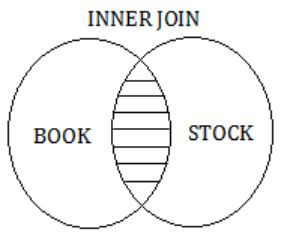So, the result of Query 1 is,Query 2:
The LEFT OUTER JOIN keyword returns all records from the left table (Book) and the matched records from the right table (Stock).
The result is NULL from the right side, if there is no match.So, the result of Query 2 is,Query 3:
The RIGHT OUTER JOIN keyword returns all records from the right table (Stock), and the matched records from the left table(BOOK).
The result is NULL from the left side, when there is no match.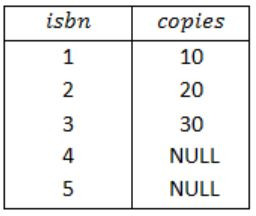Query 4:
The FULL OUTER JOIN keyword return all records when there is a match in either left (Book) or right (Stock) table records.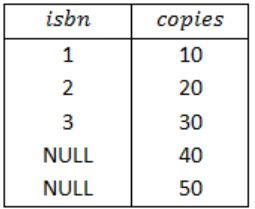So, the result of Query 4 is,Therefore, from the result of above four queries, a superset of the outputs of the Query 1, Query 2 and Query 3 is Query 4.
Note:
If we take isbn as a primary key in both the tables Book and Stock and foreign key, in one of the tables then also will get option (D) as the answer.
 Question 9

Consider the following four relational schemas. For each schema, all non-trivial functional dependencies are listed. The underlined attributes are the respective primary keys.

```Schema I: Registration(rollno, courses)
Field ‘courses’ is a set-valued attribute containing the set of
courses a student has registered for.
Non-trivial functional dependency
rollno → courses

Schema II: Registration (rollno, coursid, email)
Non-trivial functional dependencies:
rollno, courseid → email
email → rollno

Schema III: Registration (rollno, courseid, marks, grade)
Non-trivial functional dependencies:

Schema IV: Registration (rollno, courseid, credit)
Non-trivial functional dependencies:
rollno, courseid → credit
courseid → credit
```

Which one of the relational schemas above is in 3NF but not in BCNF?

 A Schema I B Schema II C Schema III D Schema IV
Database-Management-System       Normalization       GATE 2018       Video-Explanation
Question 9 Explanation:
Schema I:
Registration (rollno, courses) rollno → courses
For the given schema Registration ‘rollno’ is a primary key.
Left-side of the functional dependency is a superkey so, Registration is in BCNF.
Schema II:
Registrstion (rollno, courseid, email)
rollno, courseid → email
email → rollno
From the given schema the candidate key is (rollno + courseid).
There is no part of the key in the left hand of the FD’s so, it is in 2NF.
In the FD email→rollno, email is non-prime attribute but rollno is a prime attribute.
So, it is not a transitive dependency.
No transitive dependencies so, the schema is in 3NF.
But in the second FD email→rollno, email is not a superkey.
So, it is violating BCNF.
Hence, the schema Registration is in 3NF but not in BCNF.
Schema III:
For the schema the candidate key is (rollno + courseid).
There are no part of the keys are determining non-prime attributes.
So, the schema is in 2NF.
In the FD marks → grade, both the attributes marks and grade are non-prime.
So, it is a transitive dependency.
The FD is violating 3NF.
The schema Registration is in 2NF but not in 3NF.
Schema IV:
Registration (rollno, courseid, credit)
rollno, courseid → credit
courseid → credit
The candidate key is (rollno + courseid).
In the FD, courseid → credit, courseid is part of the key (prime attribute) and credit is non-prime.
So, it is a partial dependency.
The schema is violating 2NF.
 Question 10
Consider the relations r(A, B) and s(B, C), where s.B is a primary key and r.B is a foreign key referencing s.B. Consider the query
Q: r⋈(σ<B<5 (s))
Let LOJ denote the natural left outer-join operation. Assume that r and s contain no null values.
Which one of the following is NOT equivalent to Q?
 A σB<5 (r ⨝ s) B σB<5 (r LOJ s) C r LOJ (σB<5(s)) D σB<5(r) LOJ s
Database-Management-System       Relational-Algebra       GATE 2018       Video-Explanation
Question 10 Explanation:
Consider the following relations r(A, B) and S(B, C), where S.B is a primary key and r.B is a foreign key referencing S.B
Consider the following tables without NULL values.Q: r⨝(σB<5(S))
The result of σB<5(S) is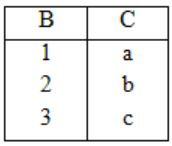The result of σB<5(S) isOption (A):
The result of r⨝S isThe result of σB<5(r⨝S) isOption (B):
The result of r LOJ S isThe result of σB<5(r LOJ S) isOption (C):
The result of σB<5(S) isNow, the result of r LOJ(σB<5(S))Option (D):
The result of σB<5(r) isNow, the result of σB<5(r) LOJ S isTherefore, from the output of above four options, the results of options, the results of options (A), (B) and (D) are equivalent to Q.
 Question 11
The following functional dependencies hold true for the relational schema {V, W, X, Y, Z} :
V → W
VW → X
Y → VX
Y → Z
Which of the following is irreducible equivalent for this set of functional dependencies?
 A V→WV→XY→VY→Z B V→WW→XY→VY→Z C V→WV→XY→VY→XY→Z D V→WW→XY→VY→XY→Z
Database-Management-System       Functional-Dependency       GATE 2017 [Set-1]       Video-Explanation
Question 11 Explanation:
Step 1:
V → W, VW → X, Y → V, Y → X, Y→ Z
Step 2:
V → W, VW → X, Y → V, Y → X, Y→ Z
(V)+ = V ×
(VW)+ = VW ×
(Y)+ = YXZ
(Y)+ = YVW ×
(Y)+ = YVWX
Without Y → X, the closure of Y is deriving ‘X’ from the remaining attributes.
So, we can remove Y → X as its redundant.
Step 3:
V → W, VW → X, Y → V, Y → Z
(V)+ = VW, the closure of V is deriving W from the remaining FD’s.
So, W is redundant. We can remove it.
So, the final canonical form is
V→W, V→X, Y→V, Y→Z
⇾ So, option (A) is correct.
 Question 12

Consider a database that has the relation schema EMP (EmpId, EmpName, and DeptName). An instance of the schema EMP and a SQL query on it are given below.The output of executing the SQL query is ___________.

 A 2.6 B 2.7 C 2.8 D 2.9
Database-Management-System       SQL       GATE 2017 [Set-1]       Video-Explanation
Question 12 Explanation:
The given query is⇾ We start evaluating from the inner query.
The inner query forms DeptName wise groups and counts the DeptName wise EmpIds.
⇾ In inner query DeptName, Count(EmpId) is the alias name DeptName, Num.
So, the output of the inner query is,The outer query will find the
Avg(Num) = (4+3+3+2+1)/5 = 2.6
 Question 13
Consider a database that has the relation schemas EMP(EmpId, EmpName, DeptId), and DEPT(DeptName, DeptId). Note that the DeptId can be permitted to a NULL in the relation EMP. Consider the following queries on the database expressed in tuple relational calculus.
(I) {t│∃u ∈ EMP(t[EmpName] = u[EmpName] ∧ ∀v ∈ DEPT(t[DeptId] ≠ v[DeptId]))}
(II) {t│∃u ∈ EMP(t[EmpName] = u[EmpName] ∧ ∃v ∈ DEPT(t[DeptId] ≠ v[DeptId]))}
(III) {t│∃u ∈ EMP(t[EmpName] = u[EmpName] ∧ ∃v ∈ DEPT(t[DeptId] = v[DeptId]))}
Which of the above queries are safe?
 A (I) and (II) only B (I) and (III) only C (II) and (III) only D (I), (II) and (III)
Database-Management-System       Relational-Algebra       GATE 2017 [Set-1]       Video-Explanation
Question 13 Explanation:
An expression is said to be safe, if it yield a finite number of tuples, otherwise unsafe.
(I) Gives EmpNames who do not belong to any Department. So, it is going to be a finite number of tuples as a result.
(II) Gives EmpNames who do not belong to some Department. This is also going to have finite number of tuples.
(III) Gives EmpNames who do not belong to same Department. This one will also give finite number of tuples.
All the expressions I, II and III are giving finite number of tuples. So, all are safe.
 Question 14
In a database system, unique timestamps are assigned to each transaction using Lamport’s logical clock. Let TS(T1) and TS(T2) be the timestamps of transactions T1 and T2 respectively. Besides, T1 holds a lock on the resource R and T2 has requested a conflicting lock on the same resource R. The following algorithm is used to prevent deadlocks in the database system assuming that a killed transaction is restarted with the same timestamp.
if TS(T2)<TS(T1) then
T1 is killed
else T2 waits.
Assume any transaction that is not killed terminates eventually. Which of the following is TRUE about the database system that uses the above algorithm to prevent deadlocks?
 A The database system is both deadlock-free and starvation-free. B The database system is deadlock-free, but not starvation-free. C The database system is starvation-free, but not deadlock-free. D The database system is neither deadlock-free nor starvation-free.
Database-Management-System       Time-stamp-Ordering       GATE 2017 [Set-1]       Video-Explanation
Question 14 Explanation:
In the question, it is given that ‘unique time stamps’ are assigned to the transactions.
So, there will be no equal timestamps.
Lamport’s logical clock:
In this timestamps are assigned in increasing order only.
According to the given algorithm,
TS(T2) < TS(T1)
then T1 is killed
else
T2 will wait
So, in both the cases, it will be deadlock free and there will be no starvation.
 Question 15

Consider a database that has the relation schema CR(StudentName, CourseName). An instance of the schema CR is as given below.The following query is made on the database.

```         T1 ← πCourseName(σStudentName='SA'(CR))
T2 ← CR ÷ T1
```

The number of rows in T2 is ____________.

 A 4 B 5 C 6 D 7
Database-Management-System       Relational-Algebra       GATE 2017 [Set-1]       Video-Explanation
Question 15 Explanation:
(1) T1 ← πCourseNameStudentName = ‘SA’(CR))
The σStudentName = ‘SA’(CR) will produce the following⇾ The result of T1 ← πCourseNameStudentName=’SA’(CR)) is(2) T2 ← CR÷T1
⇾ We see that SA is enrolled for CA, CB and CC.
⇾ T2 will give the StudentNames those who have enrolled for all the CA, C, CC courses. So, the following Students are enrolled for the given 3 courses.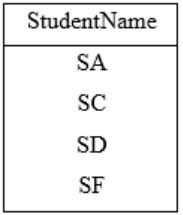⇾ So, the output of T2 will have 4 rows.
 Question 16

An ER model of a database consists of entity types A and B. These are connected by a relationship R which does not have its own attribute. Under which one of the following conditions, can the relational table for R be merged with that of A?

 A Relationship R is one-to-many and the participation of A in R is total. B Relationship R is one-to-many and the participation of A in R is partial. C Relationship R is many-to-one and the participation of A in R is total. D Relationship R is one-to-many and the participation of A in R is partial.
Database-Management-System       ER-Model       GATE 2017 [Set-2]       Video-Explanation
Question 16 Explanation:The relational table for R be merged that of A, if the relationship R is Many-to-one and the participation of A in R is total.
 Question 17

Consider the following tables T1 and T2.In table T1, P is the primary key and Q is the foreign key referencing R in table T2 with on-delete cascade and on-update cascade. In table T2, R is the primary key and S is the foreign key referencing P in table T1 with on-delete set NULL and on-update cascade. In order to delete record 〈3,8〉 from table T1, the number of additional records that need to be deleted from table T1 is _________.

 A 0 B 1 C 2 D 3
Database-Management-System       Referential-Integrity       GATE 2017 [Set-2]       Video-Explanation
Question 17 Explanation: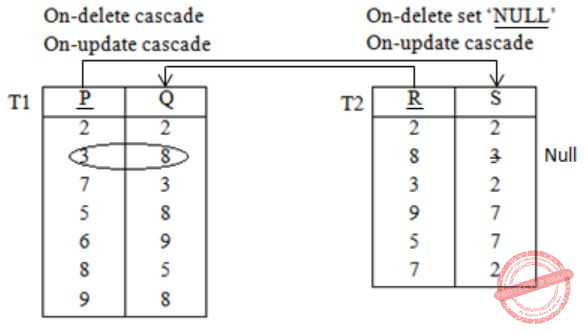Therefore, no. of additional records deleted from the table T1 is 0 (zero).
 Question 18
Two transactions T1 and T2 are given as
T1: r1(X)w1(X)r1(Y)w1(Y)
T2: r2(Y)w2(Y)r2(Z)w2(Z)
where ri(V) denotes a read operation by transaction Ti on a variable V and wi(V) denotes a write operation by transaction Ti on a variable V. The total number of conflict serializable schedules that can be formed by T1 and T2 is ____________.
 A 54 B 55 C 56 D 57
Database-Management-System       Transactions       GATE 2017 [Set-2]       Video-Explanation
Question 18 Explanation:
From the given transactions T1 and T2, total number of schedules possible = (4+4)!/4!4!
= 8!/(4×3×2×4×3×2)
= (8×7×6×5×4×3×2×1)/(4×3×2×4×3×2)
= 70
Following two conflict actions are possible: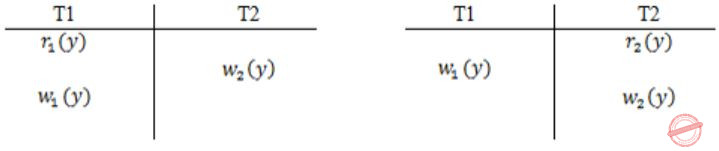∴# Permutations = 4 × 3 = 12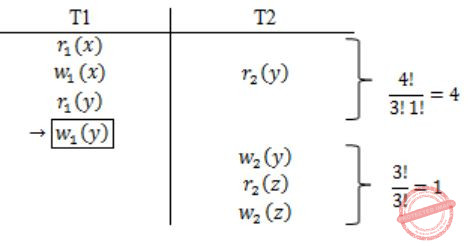#permutations = 4 × 1 = 4
∴ Total no. of conflict serial schedules possible = 70 – 12 – 4 = 54
 Question 19

Consider the following database table named top_scorer.```SELECT ta.player FROM top_scorer AS ta
WHERE ta.goals > ALL (SELECT tb.goals
FROM top_scorer AS tb
WHERE tb.country = 'Spain')
AND ta.goals > ANY (SELECT tc.goals
FROM top_scorer AS tc
WHERE tc.country = 'Germany')```

The number of tuples returned by the above SQL query is _____.

 A 7 B 8 C 9 D 10
Database-Management-System       SQL       GATE 2017 [Set-2]       Video-Explanation
Question 19 Explanation:In the given database table top_scorer no players are there from ‘Spain’.
So, the query (1) results 0 and ALL (empty) is always TRUE.
The query (2) selects the goals of the players those who are belongs to ‘Germany’.
So, it results in ANY (16, 14, 11, 10).
So, the outer most query results the player names from top_scorer, who have more goals.
Since, the minimum goal by the ‘Germany’ player is 10, it returns the following 7 rows.Question 20

Which of the following is NOT a superkey in a relational schema with attributes V, W, X, Y, Z and primary key VY?

 A VXYZ B VWXZ C VWXY D VWXYZ
Database-Management-System       Normalization       GATE 2016 [Set-1]       Video-Explanation
Question 20 Explanation:
It is given that “VY” is a primary key of the relational schema.
Any superset of “VY” is a super key. So, option (B) does not contain “Y”.
 Question 21
Which of the following is NOT a part of the ACID properties of database transactions?
 A Atomicity B Consistency C Isolation D Deadlock-freedom
Database-Management-System       Transactions       GATE 2016 [Set-1]       Video-Explanation
Question 21 Explanation:
A transaction in a database system must maintain Atomicity, Consistency, Isolation and Durability – commonly known as ACID properties.
So, Deadlock – freedom is not there in the ACID properties.
 Question 22
A database of research articles in a journal uses the following schema.(VOLUME, NUMBER, STARTPAGE, ENDPAGE, TITLE, YEAR, PRICE)
The primary key is (VOLUME, NUMBER, STARTPAGE, ENDPAGE) and the following functional dependencies exist in the schema. (VOLUME, NUMBER, STARTPAGE, ENDPAGE) → TITLE (VOLUME, NUMBER) → YEAR (VOLUME, NUMBER, STARTPAGE, ENDPAGE) → PRICE The database is redesigned to use the following schemas. (VOLUME, NUMBER, STARTPAGE, ENDPAGE, TITLE, PRICE) (VOLUME, NUMBER, YEAR) Which is the weakest normal form that the new database satisﬁes, but the old one does not?
 A 1NF B 2NF C 3NF D BCNF
Database-Management-System       Normalization       GATE 2016 [Set-1]       Video-Explanation
Question 22 Explanation:
Journal (V, N, S, E, T, Y, P)
V – VOLUME
N – NUMBER
S – STARTPAGE
E – ENDPAGE
T – TITLE
Y – YEAR
P – PRICE
Primary key: (V, N, S, E)
FD set:
(V, N, S, E) → T
(V, N) → Y
(V, N, S, E) → P
In (V, N) → Y; V, N is a part of the key and Y is non-prime attribute.
So, it is a partial dependency.
Now, the schema “Journal” is in 1NF but not in 2NF.
The database is redesigned as follows:Both R1 and R2 are in BCNF.
Therefore, 2NF is the weakest normal form that the new database satisfies, but the old one does not.
 Question 23
Consider the following two phase locking protocol. Suppose a transaction T accesses (for read or write operations), a certain set of objects{O1,…,Ok}. This is done in the following manner:
Step1. T acquires exclusive locks to O1,…,Ok in increasing order of their addresses.
Step2. The required operations are performed.
Step3. All locks are released.
This protocol will
 A guarantee serializability and deadlock-freedom B guarantee neither serializability nor deadlock-freedom C guarantee serializability but not deadlock-freedom D guarantee deadlock-freedom but not serializability
Database-Management-System       Two Phase Locking protocol       GATE 2016 [Set-1]       Video-Explanation
Question 23 Explanation:
Conservative 2PLP:
In conservative 2PL protocol, a transaction has to lock all the items before the transaction begins execution.
• Ensure serializability.
The given scenario (Step1, Step2 and Step3) is conservative 2PLP.
 Question 24

B+ Trees are considered BALANCED because

 A the lengths of the paths from the root to all leaf nodes are all equal. B the lengths of the paths from the root to all leaf nodes differ from each other by at most 1. C the number of children of any two non-leaf sibling nodes differ by at most 1. D the number of records in any two leaf nodes differ by at most 1.
Database-Management-System       B+-Trees       GATE 2016 [Set-2]       Video-Explanation
Question 24 Explanation:
In both B & B+ trees all the leaf nodes will be at same level.
 Question 25

Suppose a database schedule S involves transactions T1, …, Tn. Construct the precedence graph of S with vertices representing the transactions and edges representing the conﬂicts. If S is serializable, which one of the following orderings of the vertices of the precedence graph is guaranteed to yield a serial schedule?

 A Topological order B Depth-ﬁrst order C Breadth-ﬁrst order D Ascending order of transaction indices
Database-Management-System       Transactions       GATE 2016 [Set-2]       Video-Explanation
Question 25 Explanation:
If a schedule is conflict serializable then no cycle in precedence graph should be present.
But BFS and DFS are also possible for cyclic graphs.
And topological sort is not possible for cyclic graph.
Moreover option (D) is also wrong because in a transaction with more indices might come before lower one.
 Question 26
Consider the following database schedule with two transactions, T1 and T2.
S = r2(X); r1(X); r2(Y); w1(X); r1(Y); w2(X); a1; a2
where ri(Z) denotes a read operation by transaction Ti on a variable Z, wi(Z) denotes a write operation by Ti on a variable Z and ai denotes an abort by transaction Ti.
Which one of the following statements about the above schedule is TRUE?
 A S is non-recoverable B S is recoverable, but has a cascading abort C S does not have a cascading abort D S is strict
Database-Management-System       Transactions       GATE 2016 [Set-2]       Video-Explanation
Question 26 Explanation:
Given schedule is,Now let’s check statements one by one,
A) False, because there is no dirty read. So, it is recoverable.
B) False, because there is to dirty read. So, no cascading aborts.
C) True.
D) False, because there is Transaction T2 which written the value of x which is written by T1 before T1 has aborted. So, not strict.
 Question 27

Consider the following database table named water_schemes :The number of tuples returned by the following SQL query is _________.

```with total(name, capacity) as
select district_name, sum(capacity)
from water_schemes
group by district_name
with total_avg(capacity) as
select avg(capacity)
from total
select name
from total, total_avg
where total.capacity ≥ total_avg.capacity
```
 A 2 B 3 C 4 D 5
Database-Management-System       SQL       GATE 2016 [Set-2]       Video-Explanation
Question 27 Explanation:
• The SQL WITH clause allows you to give a sub-query block a name (a process also called sub-query refactoring), which can be referenced in several places within the main SQL query.
The name assigned to the sub-query is treated as though it was an inline view or table.
• First group by district name is performed and total capacities are obtained as following:• Then average capacity is computed,
Average Capacity = (20 + 40 + 30 + 10)/4
= 100/4
= 25
• Finally, 3rd query will be executed and it’s tuples will be considered as output, where name of district and its total capacity should be more than or equal to 25.
• Then average capacity is computed,
Average Capacity = (20 + 40 + 30 + 10)/4
= 100/4
= 25
• Finally, 3rd query will be executed and it’s tuples will be considered as output, where name of district and its total capacity should be more than or equal to 25.Question 28

SELECT operation in SQL is equivalent to

 A the selection operation in relational algebra B the selection operation in relational algebra, except that SELECT in SQL retains duplicates C the projection operation in relational algebra D the projection operation in relational algebra, except that SELECT in SQL retains duplicates
Database-Management-System       SQL       GATE 2015 [Set-1]
Question 28 Explanation:
SELECT operation in SQL perform vertical partitioning which is performed by projection operation in relational calculus but SQL is multi sets; hence (D).
 Question 29

Consider an Entity-Relationship (ER) model in which entity sets E1 and E2 are connected by an m:n relationship R12, E1 and E3 are connected by a 1:n (1 on the side of E1 and n on the side of E3) relationship R13.

E1 has two single-valued attributes a11 and a12 of which a11 is the key attribute. E2 has two single-valued attributes a21 and a22 is the key attribute. E3 has two single-valued attributes a31 and a32 of which a31 is the key attribute. The relationships do not have any attributes.

If a relational model is derived from the above ER model, then the minimum number of relations that would be generated if all the relations are in 3NF is ___________.

 A 4 B 6 C 7 D 8
Database-Management-System       ER-Model       GATE 2015 [Set-1]
Question 29 Explanation: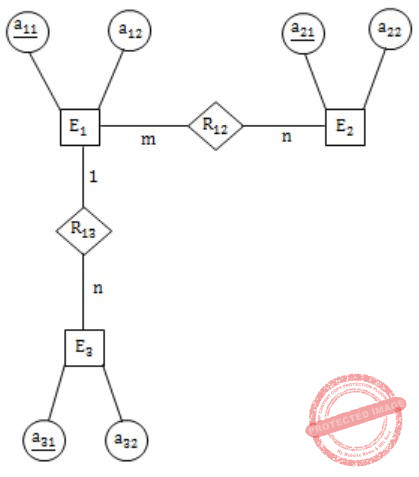Question 30

Consider the following relations:Consider the following SQL query.

```SELECT S. Student_Name, sum(P.Marks)
FROM Student S, Performance P
WHERE S.Roll_No = P.Roll_No
GROUP BY S.Student_Name```

The number of rows that will be returned by the SQL query is _________.

 A 2 B 3 C 4 D 5
Database-Management-System       SQL       GATE 2015 [Set-1]
Question 30 Explanation:
Output table isQuestion 31

Consider the following transaction involving two bank account x and y.

The constraint that the sum of the accounts x and y should remain constant is that of

 A Atomicity B Consistency C Isolation D Durability
Database-Management-System       Transactions       GATE 2015 [Set-2]
Question 31 Explanation:
The consistency property ensures that the database remains in a consistent state before the (start of the transaction and after the transaction is over. Here sum of the accounts x & y should remain same before & after execution of the given transactions which refers to the consistency of the sum.
 Question 32

With reference to the B+ tree index of order 1 shown below, the minimum number of nodes (including the Root node) that must be fetched in order to satisfy the following query:”Get all records with a search key grater than or equal to 7 and less than 15″ is ___________.A 6 B 7 C 5 D 9
Database-Management-System       B+-Trees       GATE 2015 [Set-2]
Question 32 Explanation:Question 33

Consider two relations R1(A,B) with the tuples (1,5), (3,7) and R2(A,C) = (1,7), (4,9). Assume that R(A,B,C) is the full natural outer join of R1 and R2. Consider the following tuples of the form (A,B,C): a = (1.5,null), b = (1,null,7), c = (3,null,9), d = (4,7,null), e = (1,5,7), f = (3,7,null), g = (4,null,9). Which one of the following statements is correct?

 A R contains a,b,e,f,g but not c, d. B R contains all of a,b,c,d,e,f,g C R contains e,f,g but not a,b D R contains e but not f,g
Database-Management-System       Relational-Algebra       GATE 2015 [Set-2]
Question 33 Explanation:⋆ So, from the above resultant table, R contains e, f, g only but not a, b.
 Question 34

Consider a simple checkpointing protocol and the following set of operations in the log.

(start, T4); (write, T4, y, 2, 3); (start, T1); (commit, T4); (write, T1, z, 5, 7);
(checkpoint);
(start, T2); (write, T2, x, 1, 9); (commit, T2); (start, T3); (write, T3, z, 7, 2);

If a crash happens now and the system tries to recover using both undo and redo operations, what are the contents of the undo list and the redo list

 A Undo T3, T1; Redo T2 B Undo T3, T1; Redo T2, T4 C Undo: none; redo: T2, T4, T3, T1 D Undo T3, T1; T4; Redo: T2
Database-Management-System       Transactions       GATE 2015 [Set-2]
Question 34 Explanation:
As T1 & T3 are not yet committed they must be undone. The transactions which are after the latest checkpoint must be redone. So T2 must be redone. No need to redo the records which are before the last checkpoint, so T4 need not be redone.
 Question 35

Consider the relation X(P, Q, R, S, T, U) with the following set of functional dependencies

```F = {
{P, R} → {S,T},
{P, S, U} → {Q, R}
}```

Which of the following is the trivial functional dependency in F+ is closure of F?

 A {P,R}→{S,T} B {P,R}→{R,T} C {P,S}→{S} D {P,S,U}→{Q}
Database-Management-System       Functional-Dependency       GATE 2015 [Set-3]
Question 35 Explanation:
X→Y is trivial if Y⊆ X
 Question 36

Consider the following relation

`  Cinema (theater, address, capacity)`

Which of the following options will be needed at the end of the SQL query

```SELECT P1. address
FROM Cinema P1```

Such that it always finds the addresses of theaters with maximum capacity?

 A WHERE P1.capacity >= All (select P2.capacity from Cinema P2) B WHERE P1.capacity >= Any (select P2.capacity from Cinema P2) C WHERE P1.capacity > All (select max(P2.capacity) from Cinema P2) D WHERE P1.capacity > Any (select max(P2.capacity) from Cinema P2)
Database-Management-System       SQL       GATE 2015 [Set-3]
Question 36 Explanation:
Inner query collects capacities of all the theaters and in outer query we are filtering the tuples with the condition “capacity >= All”.
So the theaters which are having maximum capacity will satisfy the condition.
 Question 37

The value ofis

 A 0 B 1/2 C 1 D ∞
Database-Management-System       Calculus       GATE 2015 [Set-3]
Question 37 Explanation:Question 38

Suppose Xi for i = 1, 2, 3 are independent and identically distributed random variables whose probability mass functions are Pr[Xi = 0] = Pr[Xi = 1]=1/2 for i = 1, 2, 3. Define another random variable Y = X1X2 ⊕ X3, where ⊕ denotes XOR. Then Pr[Y = 0|X3 = 0] = _______________.

 A 0.75 B 0.76 C 0.77 D 0.78
Database-Management-System       Probability       GATE 2015 [Set-3]
Question 38 Explanation: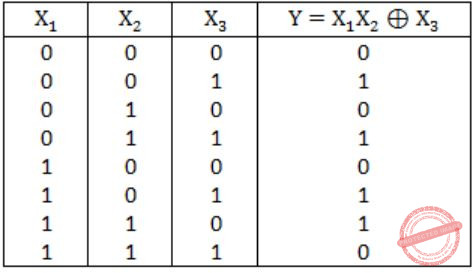Pr(Y=0/ X3=0) = Pr(Y=0 ∩ X3=0)/ Pr(X3=0)
= 3/8 / 4/8 = 3/4 = 0.75
 Question 39

Consider the relation scheme R = (E, F, G, H, I, J, K, L, M, N) and the set of functional dependencies  {{E,F} → {G}, {F} → {I,J}, {E,H} → {K,L}, {K} → {M}, {L} → {N}} on R. What is the key for R?

 A {E,F} B {E,F,H} C {E,F,H,K,L} D {E}
Database-Management-System       Functional-Dependency       GATE 2014 [Set-1]
Question 39 Explanation:
A) (EF)+ = EFGIJ. So, EF is not a key.
B) (EFH)+ = EFHGIJKLMN, EFH is deriving all the attributes of R. So, EFH is a key.
C) If EFH is a key, then EFHKL will become the super key.
D) (E)+ = E
 Question 40

Given the following statements:

```    S1: A foreign key declaration can always
be replaced by an equivalent check
assertion in SQL.
S2: Given the table R(a,b,c) where a and
b together form the primary key, the
following is a valid table definition.
CREATE TABLE S (
a INTEGER,
d INTEGER,
e INTEGER,
PRIMARY KEY (d),
FOREIGN KEY (a) references R)```

Which one of the following statements is CORRECT?

 A S1 is TRUE and S2 is FALSE. B Both S1 and S2 are TRUE. C S1 is FALSE and S2 is TRUE. D Both S1 and S2 are FALSE.
Database-Management-System       SQL       GATE 2014 [Set-1]
Question 40 Explanation:
S1: False
Using a check constraint, we can have the same effect as foreign key while adding elements to the child table. But while deleting elements from the parent table the referential integrity constraint is no longer valid. So, a check constraint cannot replace a foreign key.
S2: False:
Foreign key in one table should be defined as a primary key in other table. In above table definition, table S has a foreign key that refers to field ‘a’ of R. The field ‘a’ in table S is part of the primary key and part of the key cannot be declared as a foreign key.
 Question 41

Consider the following four schedules due to three transactions (indicated by the subscript) using read and write on a data item x, denoted by r(x) and w(x) respectively. Which one of them is conflict serializable?

 A r1 (x); r2 (x); w1 (x); r3 (x); w2 (x) B r2 (x);r1 (x);w2 (x);r3 (x);w1 (x) C r3 (x);r2 (x);r1 (x);w2 (x);w1 (x) D r2 (x);w2 (x);r3 (x);r1 (x);w1 (x)
Database-Management-System       Transactions       GATE 2014 [Set-1]
Question 41 Explanation:
Option: A– Polygraph contains cycle. So, not a conflict serializable.
Option: B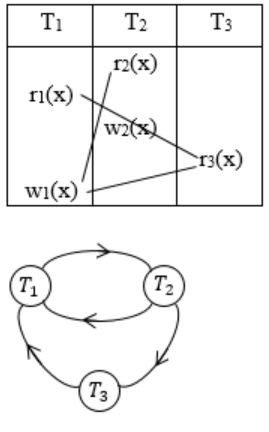-Cyclic
Option: C– Cyclic
Option: D– Acyclic, so conflict serializable.
 Question 42

Given the following two statements:

```
S1: Every table with two single-valued
attributes is in 1NF, 2NF, 3NF and BCNF.

S2: AB->C, D->E, E->C is a minimal cover
for the set of functional dependencies
AB->C, D->E, AB->E, E->C.```

Which one of the following is CORRECT?

 A S1 is TRUE and S2 is FALSE. B Both S1 and S2 are TRUE. C S1 is FALSE and S2 is TRUE. D Both S1 and S2 are FALSE.
Database-Management-System       Normalization       GATE 2014 [Set-1]
Question 42 Explanation:
S1: True
If we can prove the relation is in BCNF then by default it would be in 1NF, 2NF, 3NF also.
Let R(AB) be a two attribute relation, then
If {A→B} exists then BCNF since {A}+ = AB = R
If {B→A} exists then BCNF since {B}+ = AB = R
If {A→B, B→A} exists then BCNF since A and B both are Super Key now.
If {No non-trivial Functional Dependency} then default BCNF.
Hence it’s proved that a Relation with two single-valued attributes is in BCNF hence it’s also in 1NF, 2NF, 3NF.
S2: False
The canonical cover for the given FD set is {AB→C, D→E, AB→E, E→C}. As we can see AB→E is not covered in minimal cover since {AB}+ = ABC in the given cover {AB→C, D→E, E→C}
 Question 43

Given the following schema:

```     employees(emp-id, first-name, last-name, hire-date, dept-id, salary)
departments(dept-id, dept-name, manager-id, location-id)```

You want to display the last names and hire dates of all latest hires in their respective departments in the location ID 1700. You issue the following query:

```SQL> SELECT last-name, hire-date
FROM employees
WHERE (dept-id, hire-date) IN ( SELECT dept-id, MAX(hire-date)
FROM employees JOIN departments USING(dept-id)
WHERE location-id = 1700
GROUP BY dept-id); ```

What is the outcome?

 A It executes but does not give the correct result. B It executes and gives the correct result. C It generates an error because of pairwise comparison. D It generates an error because the GROUP BY clause cannot be used with table joins in a subquery.
Database-Management-System       SQL       GATE 2014 [Set-1]
Question 43 Explanation:
The given SQL query will display the last names and hire-dates of all latest hires in their respective departments in the location ID 1700. So, correct option is (B).
 Question 44

The maximum number of superkeys for the relation schema R(E, F, G, H) with E as the key is _____.

 A 8 B 9 C 10 D 11
Database-Management-System       Candidate-Keys       GATE 2014 [Set-2]
Question 44 Explanation:
Maximum no. of possible super keys for a relation with n attributes with one candidate key (only one attribute) = 2n-1
Here, n = 4.
So, the possible super keys = 24-1 = 8
The super keys are: E, EH, EG, EF, EGH, EFH, EFG, EFGH.
 Question 45

Given the STUDENTS relation as shown below.For (StudentName, StudentAge) to be the key for this instance, the value X should not be equal to

 A 19 B 20 C 21 D 22
Database-Management-System       Candidate-Keys       GATE 2014 [Set-2]
Question 45 Explanation:
There is already one entry with StudentName as “Shankar” and Age as 19. So, X value should not be 19.
 Question 46

Consider the following schedule S of transactions T1, T2, T3, T4:Which one of the following statements is CORRECT?

 A S is conflict-serializable but not recoverable B S is not conflict-serializable but is recoverable C S is both conflict-serializable and recoverable D S is neither conflict-serializable nor is it recoverable
Database-Management-System       Transactions       GATE 2014 [Set-2]
Question 46 Explanation:In the precedence graph, there are no cycles. So, it is conflict serializable and recoverable also.
 Question 47

Consider a join (relation algebra) between relations r(R) and s(S) using the nested loop method. There are 3 buffers each of size equal to disk block size, out of which one buffer is reserved for intermediate results. Assuming size(r(R)) < size(s(S)), the join will have fewer number of disk block accesses if

 A relation r(R) is in the outer loop. B relation s(S) is in the outer loop. C join selection factor between r(R) and s(S) is more than 0.5. D join selection factor between r(R) and s(S) is less than 0.5.
Database-Management-System       Joins       GATE 2014 [Set-2]
Question 47 Explanation:
The join between r(R) and s(S) which is using nested loop will be as follows,
For each tuple r in R do
For each tuple s in S do
If r and s satisfy the join condition then
output the tuple
The above algorithm involves (nr*bs+br)
block transfer and (nr+br) seeks.
where,
br → no. of blocks in R
bs → no. of blocks in S
hr → no. of tuples in R
To have less block accesses in nr should be less and in question it is given that size(r(R)) < size(s(S)).
To have fewer no. of disk block accesses the relation r(R) should be in outer loop.
 Question 48

SQL allows tuples in relations, and correspondingly defines the multiplicity of tuples in the result of joins. Which one of the following queries always gives the same answer as the nested query shown below:

`    select * from R where a in (select S.a from S)`
 A select R.* from R,S where R.a=S.a B select distinct R.* from R,S where R.a=S.a C select R.* from R,(select distinct a from S) as S1 where R.a=S1.a D select R.* from R,S where R.a=S.a and is unique R
Database-Management-System       SQL       GATE 2014 [Set-2]
Question 48 Explanation:
Multiplicity of duplicate tuples will be distributed when there is a match between R.a and S.a; and for that match, S.a’s value is repeated in each cases except the third case. So, the output of query given in the question matches with the output of (C).
 Question 49

What is the optimized version of the relation algebra expression πA1A2F1F2(r)))), where A1, A2 are sets of attributes in  with A1 ⊂ A2 and F1, F2 are Boolean expressions based on the attributes in r?

 A πA1 (σ(F1∧F2) (r)) B πA1 (σ(F1∨F2) (r)) C πA2 (σ(F1∧F2) (r)) D πA2 (σ(F1∨F2) (r))
Database-Management-System       ER-Model       GATE 2014 [Set-3]
Question 49 Explanation:
 Since A1 ⊂ A2 will get only attribute A1 as it is in the outside. So we can remove project A2.
 Two Selects with Boolean expression can be combined into one select with AND of two Boolean expressions.
 Question 50

A prime attribute of a relation scheme is an attribute that appears

 A in all candidate keys of R. B in some candidate key of R. C in a foreign key of R. D only in the primary key of R .
Database-Management-System       ER-Model       GATE 2014 [Set-3]
Question 50 Explanation:
A prime attribute of a relation scheme R is an attribute that appears in some candidate keys of R. Need not to appear in all the candidate keys.
Ex: AB, BC, CD are candidate keys of R(ABCD). In the FDs set one attribute may not be part of all the FDs.
There are 50 questions to complete.

Register Now